# Systems of equations Systems of equations Solving for

• Slides: 22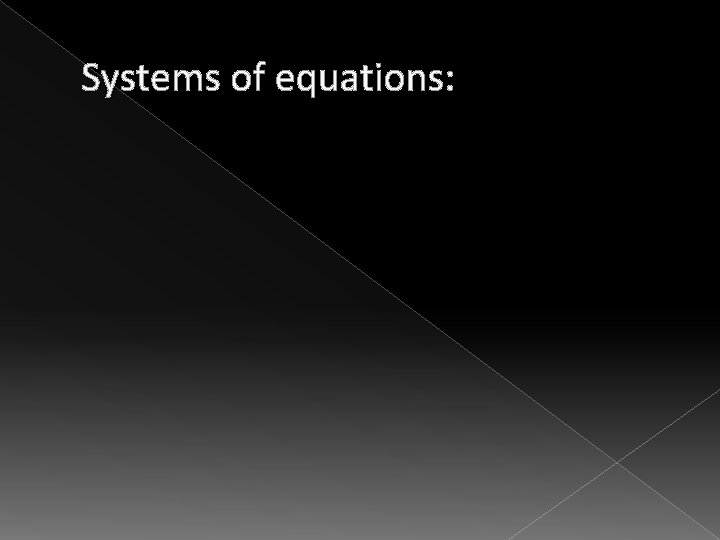Systems of equations:Systems of equations: � Solving for systems of equations: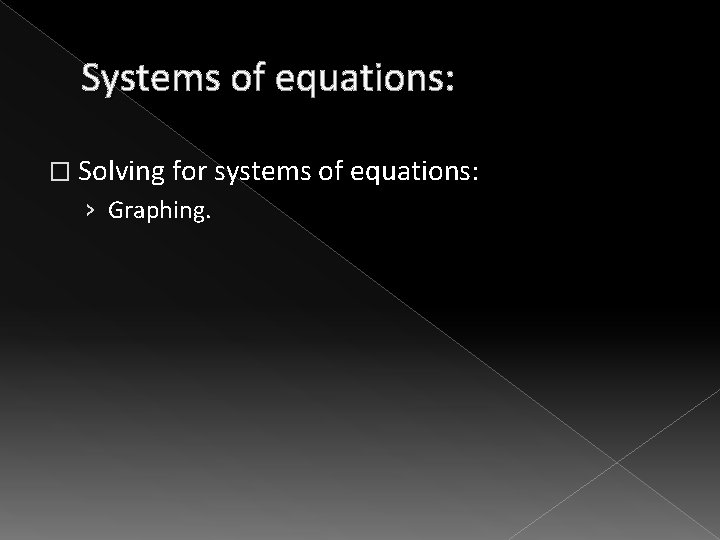Systems of equations: � Solving for systems of equations: › Graphing.Systems of equations: � Solving for systems of equations: › Graphing. �In order to solve by graphing you have to get the equation in slope-intercept form.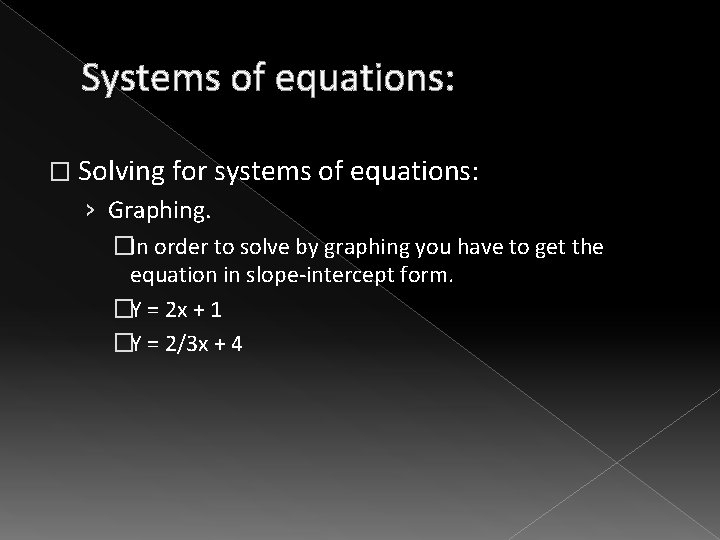Systems of equations: � Solving for systems of equations: › Graphing. �In order to solve by graphing you have to get the equation in slope-intercept form. �Y = 2 x + 1 �Y = 2/3 x + 4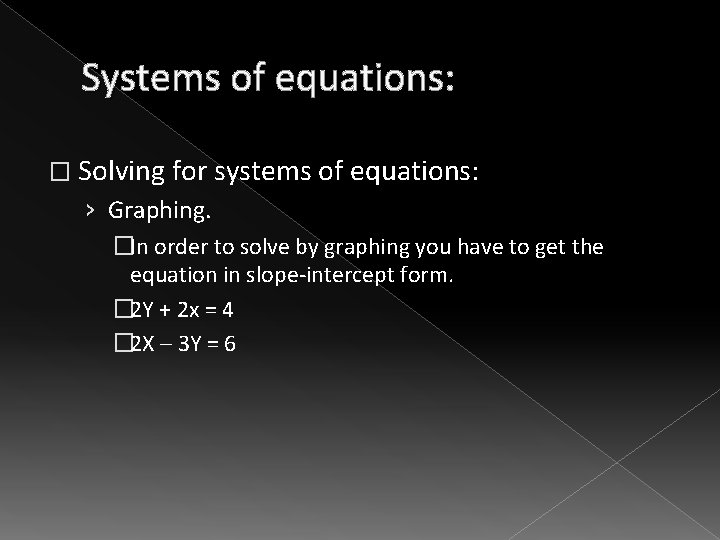Systems of equations: � Solving for systems of equations: › Graphing. �In order to solve by graphing you have to get the equation in slope-intercept form. � 2 Y + 2 x = 4 � 2 X – 3 Y = 6Systems of equations: � Solving for systems of equations: › Graphing. �In order to solve by graphing you have to get the equation in slope-intercept form. � 2 Y + 2 x = 4 � 2 X – 3 Y = 6 › When the equation is in this form we can get the answer from our calculator.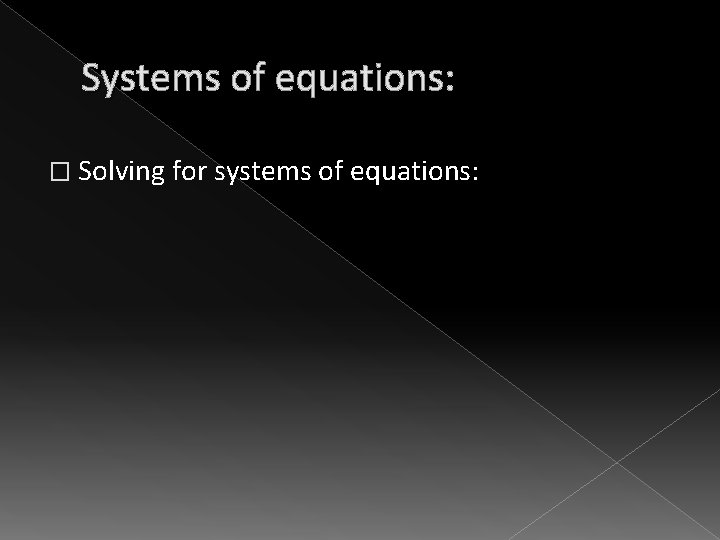Systems of equations: � Solving for systems of equations:Systems of equations: � Solving for systems of equations: › Elimination: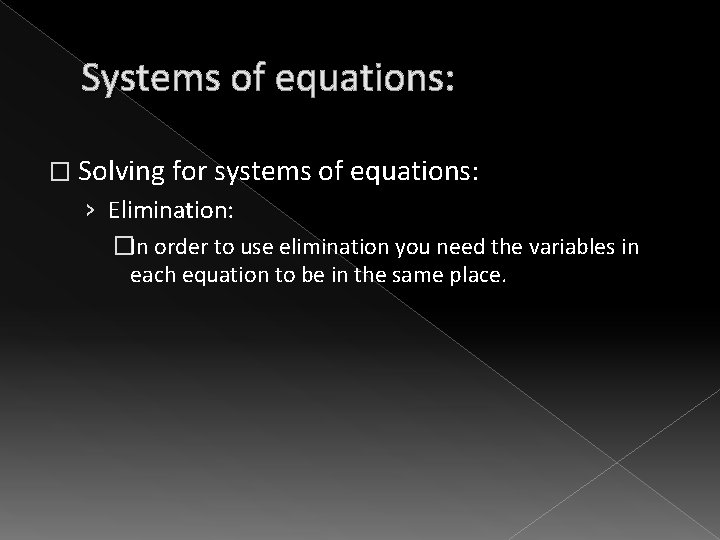Systems of equations: � Solving for systems of equations: › Elimination: �In order to use elimination you need the variables in each equation to be in the same place.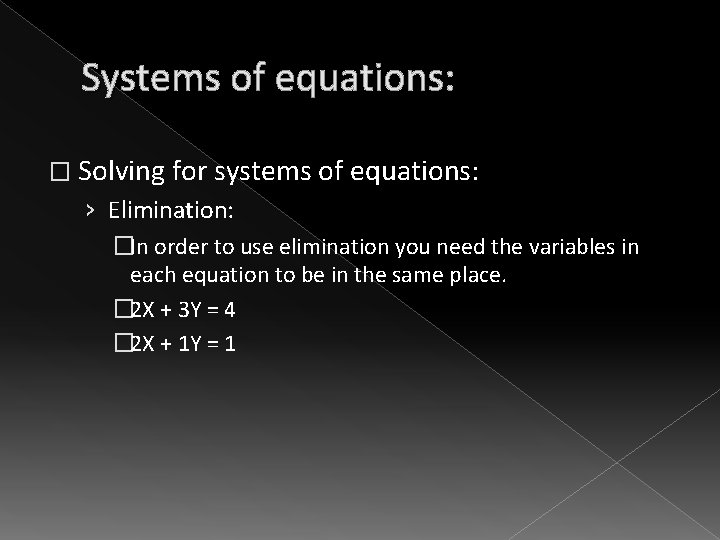Systems of equations: � Solving for systems of equations: › Elimination: �In order to use elimination you need the variables in each equation to be in the same place. � 2 X + 3 Y = 4 � 2 X + 1 Y = 1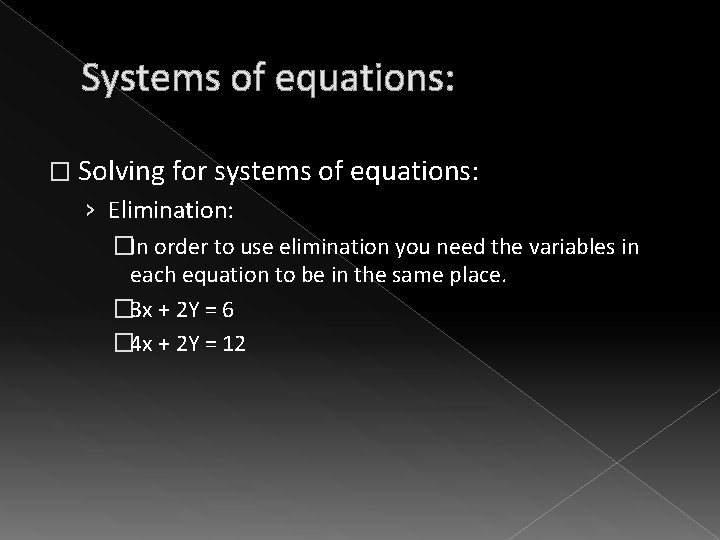Systems of equations: � Solving for systems of equations: › Elimination: �In order to use elimination you need the variables in each equation to be in the same place. � 3 x + 2 Y = 6 � 4 x + 2 Y = 12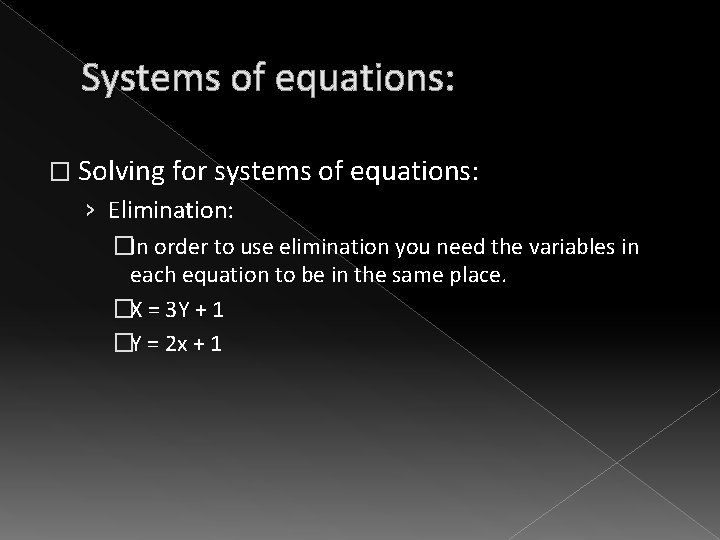Systems of equations: � Solving for systems of equations: › Elimination: �In order to use elimination you need the variables in each equation to be in the same place. �X = 3 Y + 1 �Y = 2 x + 1Systems of equations: � Solving for systems of equations: › Substitution: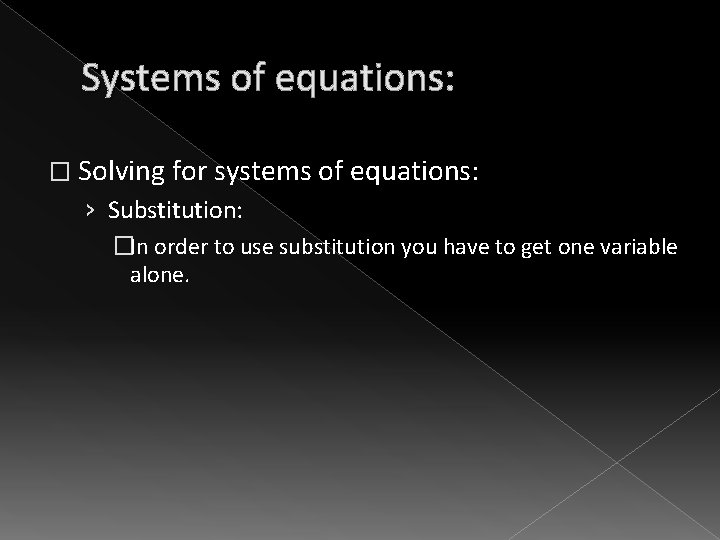Systems of equations: � Solving for systems of equations: › Substitution: �In order to use substitution you have to get one variable alone.Systems of equations: � Solving for systems of equations: › Substitution: �In order to use substitution you have to get one variable alone. �Y = 3 x + 1 �Y = 2 x + 4Systems of equations: � Solving for systems of equations: › Substitution: �In order to use substitution you have to get one variable alone. �Y = 3 x + 1 � 2 x + Y = 4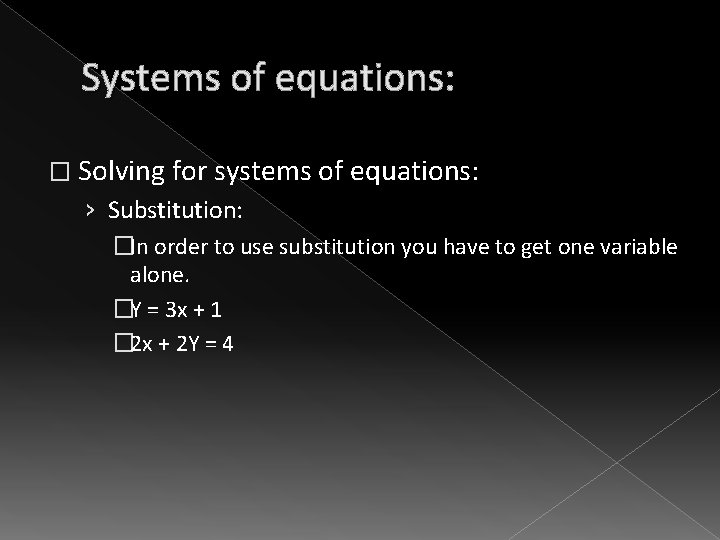Systems of equations: � Solving for systems of equations: › Substitution: �In order to use substitution you have to get one variable alone. �Y = 3 x + 1 � 2 x + 2 Y = 4Systems of equations: � Solving for systems of equations: › Substitution: �In order to use substitution you have to get one variable alone. �Y = 3 x + 1 � 2 x - 2 Y = 4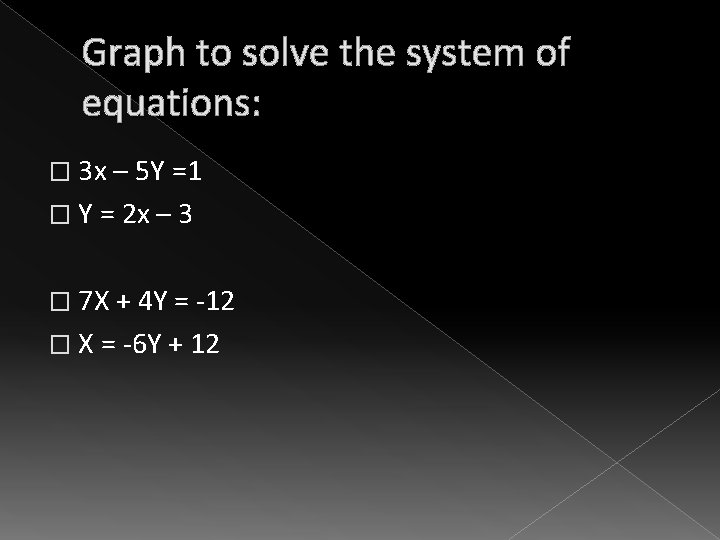Graph to solve the system of equations: � 3 x – 5 Y =1 � Y = 2 x – 3 � 7 X + 4 Y = -12 � X = -6 Y + 12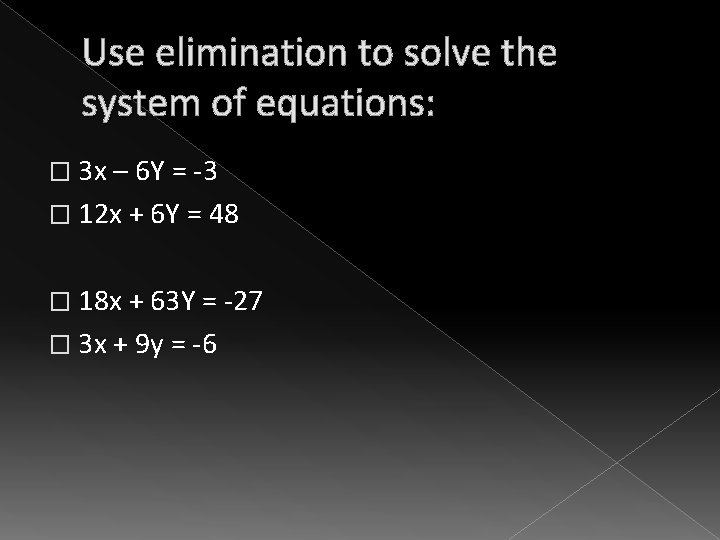Use elimination to solve the system of equations: � 3 x – 6 Y = -3 � 12 x + 6 Y = 48 � 18 x + 63 Y = -27 � 3 x + 9 y = -6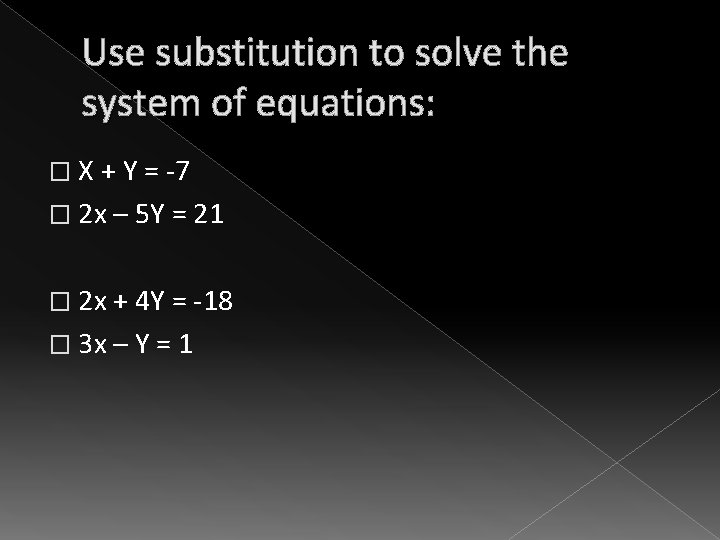Use substitution to solve the system of equations: � X + Y = -7 � 2 x – 5 Y = 21 � 2 x + 4 Y = -18 � 3 x – Y = 1